# 15 Memory

## 15.1 Object size

1. Q: Repeat the analysis above for numeric, logical, and complex vectors.

A:

numeric:

library(pryr)
sizes <- sapply(0:50, function(n) object_size(vector("numeric", n)))
plot(0:50, sizes, xlab = "Length", ylab = "Size (bytes)",
type = "s")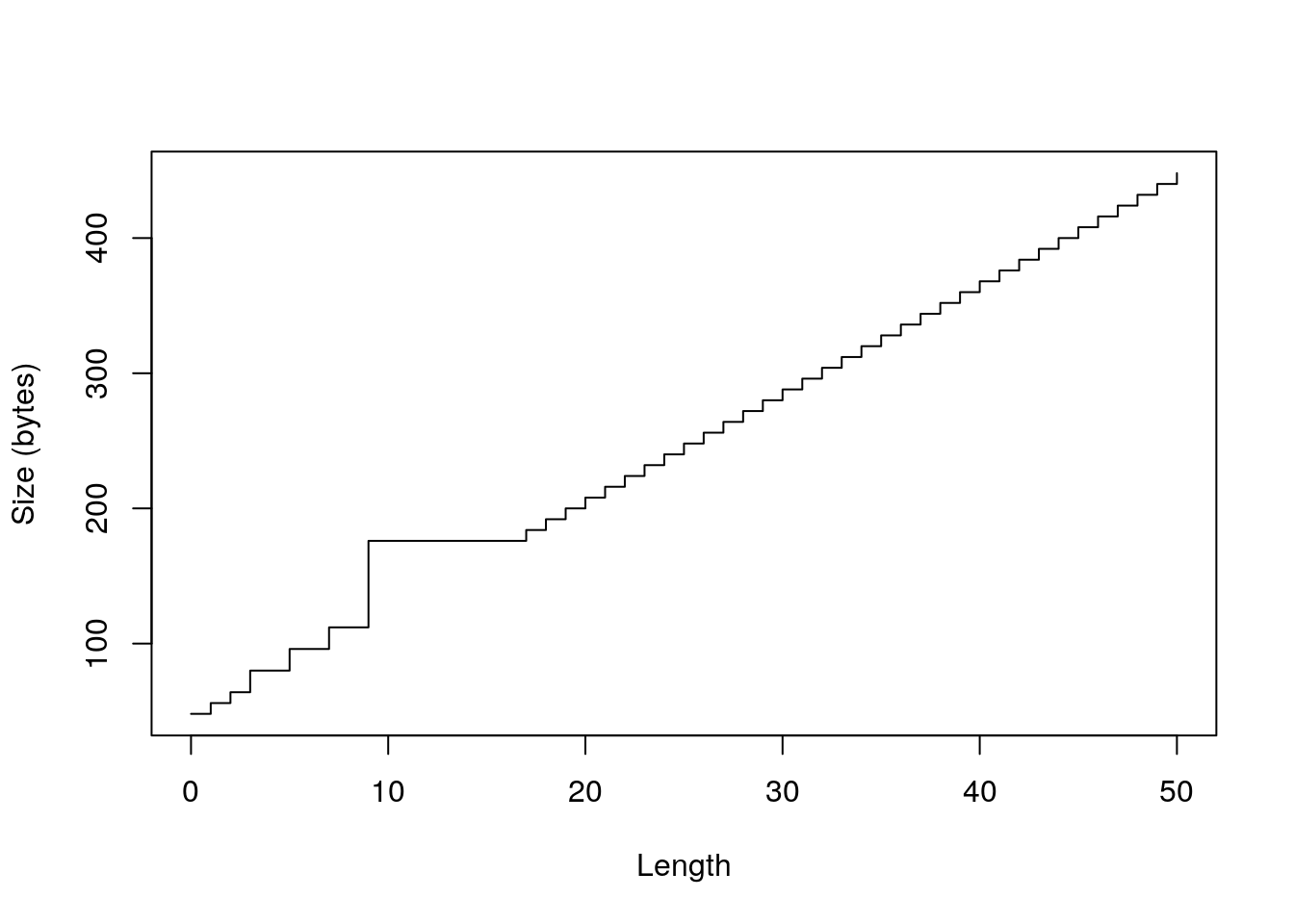plot(0:50, sizes - 40, xlab = "Length",
ylab = "Bytes excluding overhead", type = "n")
abline(h = 0, col = "grey80")
abline(h = c(8, 16, 32, 48, 64, 128), col = "grey80")
abline(a = 0, b = 8, col = "grey90", lwd = 4)
lines(sizes - 40, type = "s")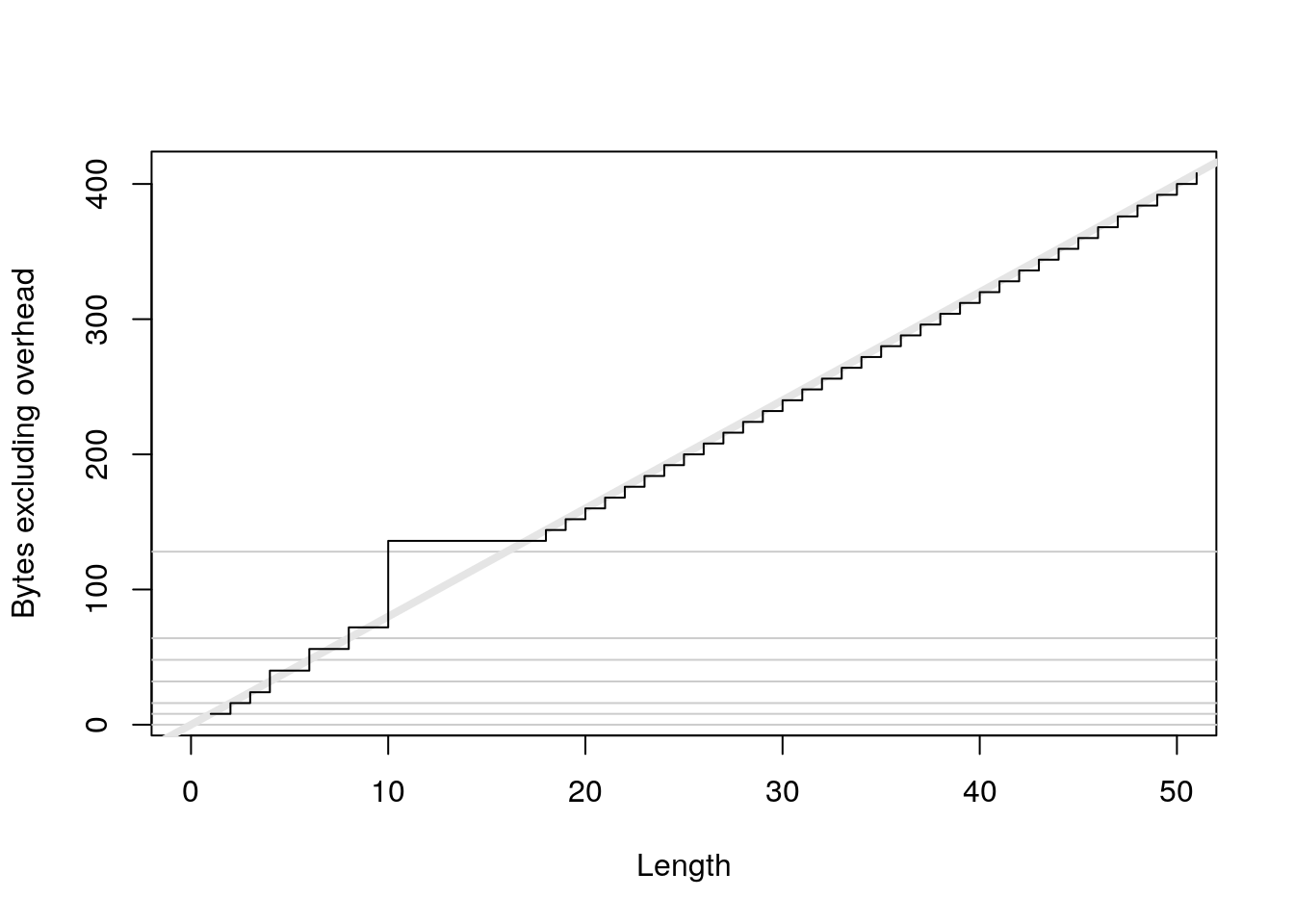x <- numeric(1e6)
object_size(x)
#> 8 MB
y <- list(x, x, x)
object_size(y)
#> 8 MB

logical:

sizes <- sapply(0:50, function(n) object_size(vector("logical", n)))
plot(0:50, sizes, xlab = "Length", ylab = "Size (bytes)",
type = "s")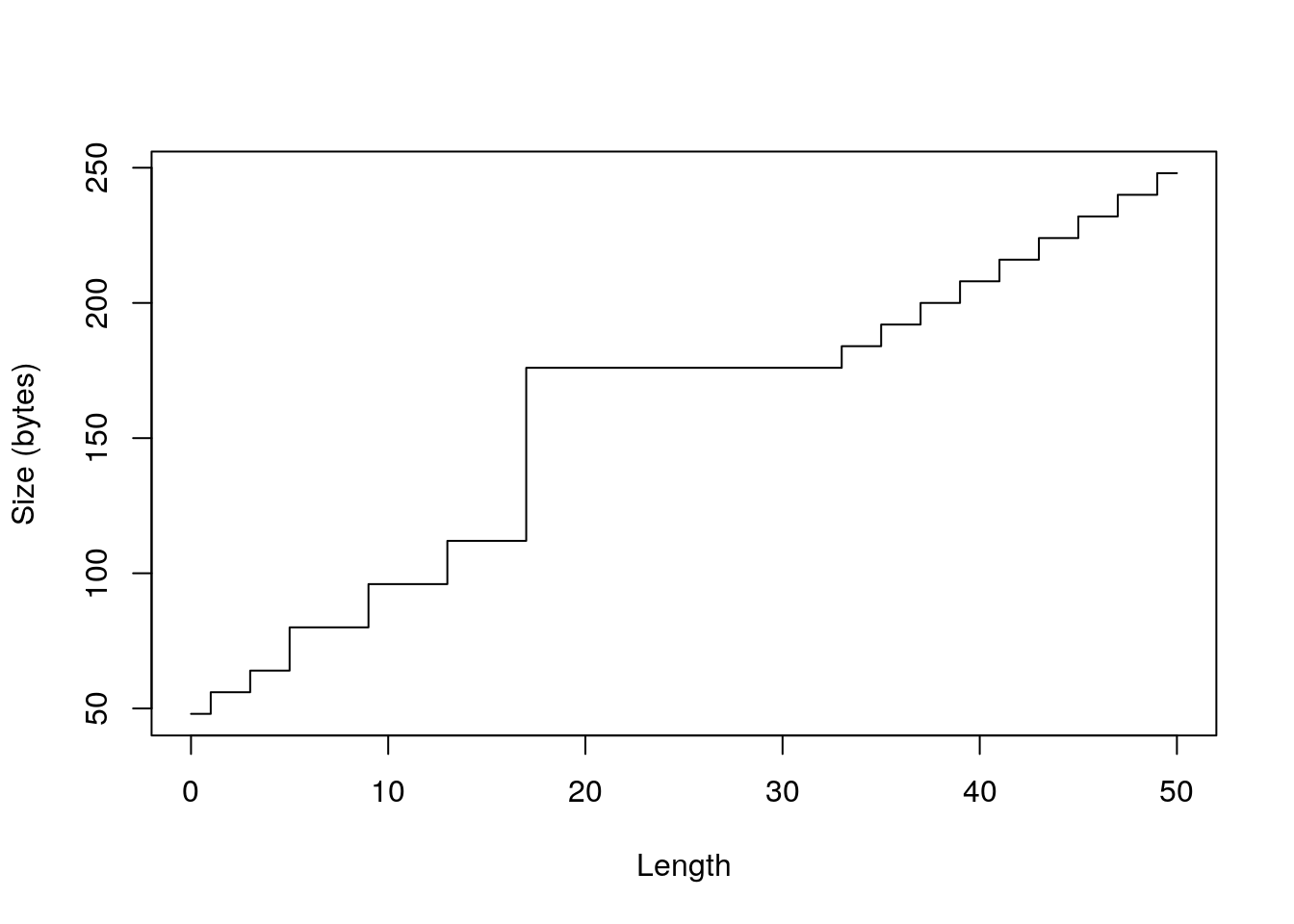plot(0:50, sizes - 40, xlab = "Length",
ylab = "Bytes excluding overhead", type = "n")
abline(h = 0, col = "grey80")
abline(h = c(8, 16, 32, 48, 64, 128), col = "grey80")
abline(a = 0, b = 4, col = "grey90", lwd = 4)
lines(sizes - 40, type = "s")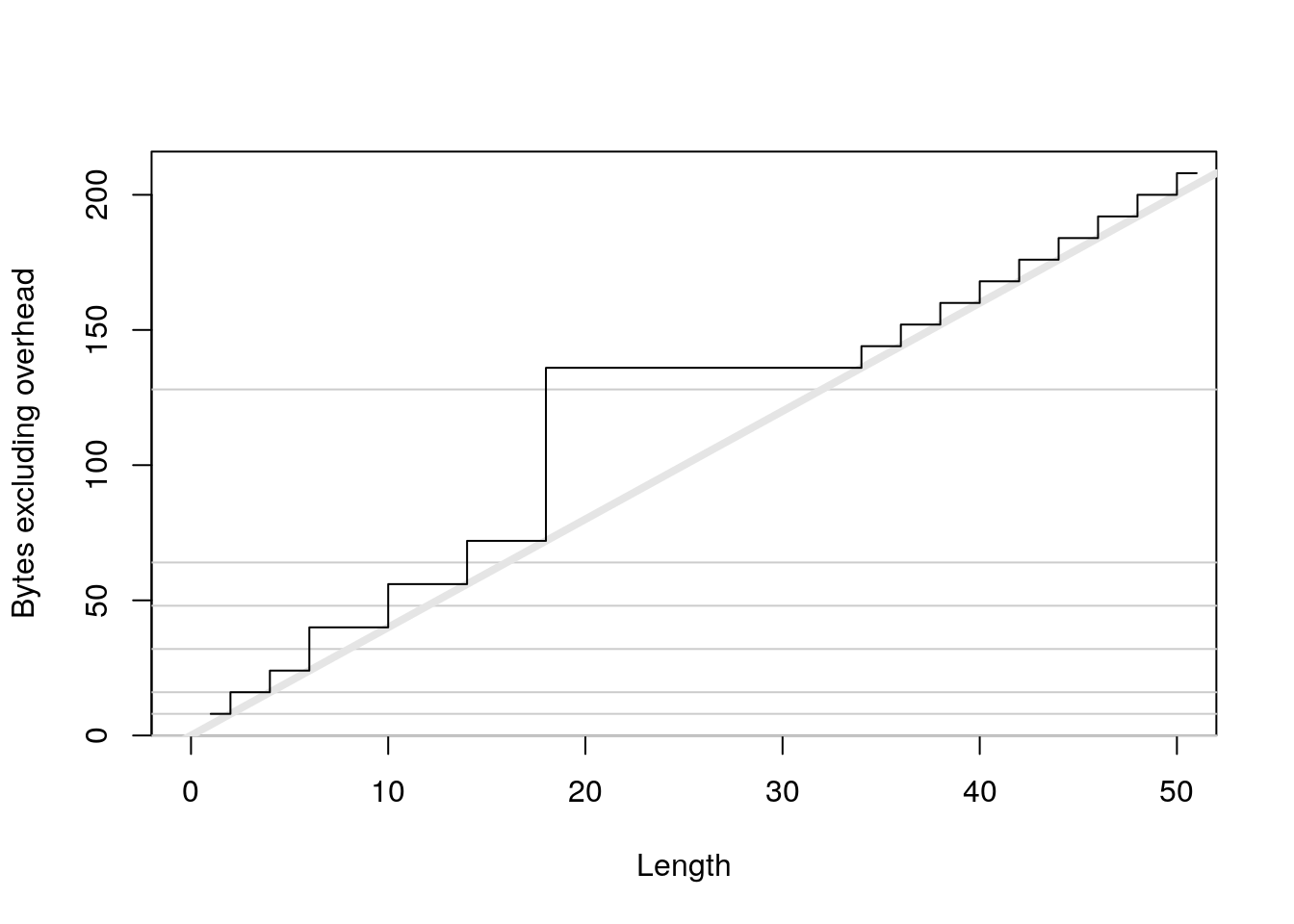x <- logical(1e6)
object_size(x)
#> 4 MB
y <- list(x, x, x)
object_size(y)
#> 4 MB

complex:

sizes <- sapply(0:50, function(n) object_size(vector("complex", n)))
plot(0:50, sizes, xlab = "Length", ylab = "Size (bytes)",
type = "s")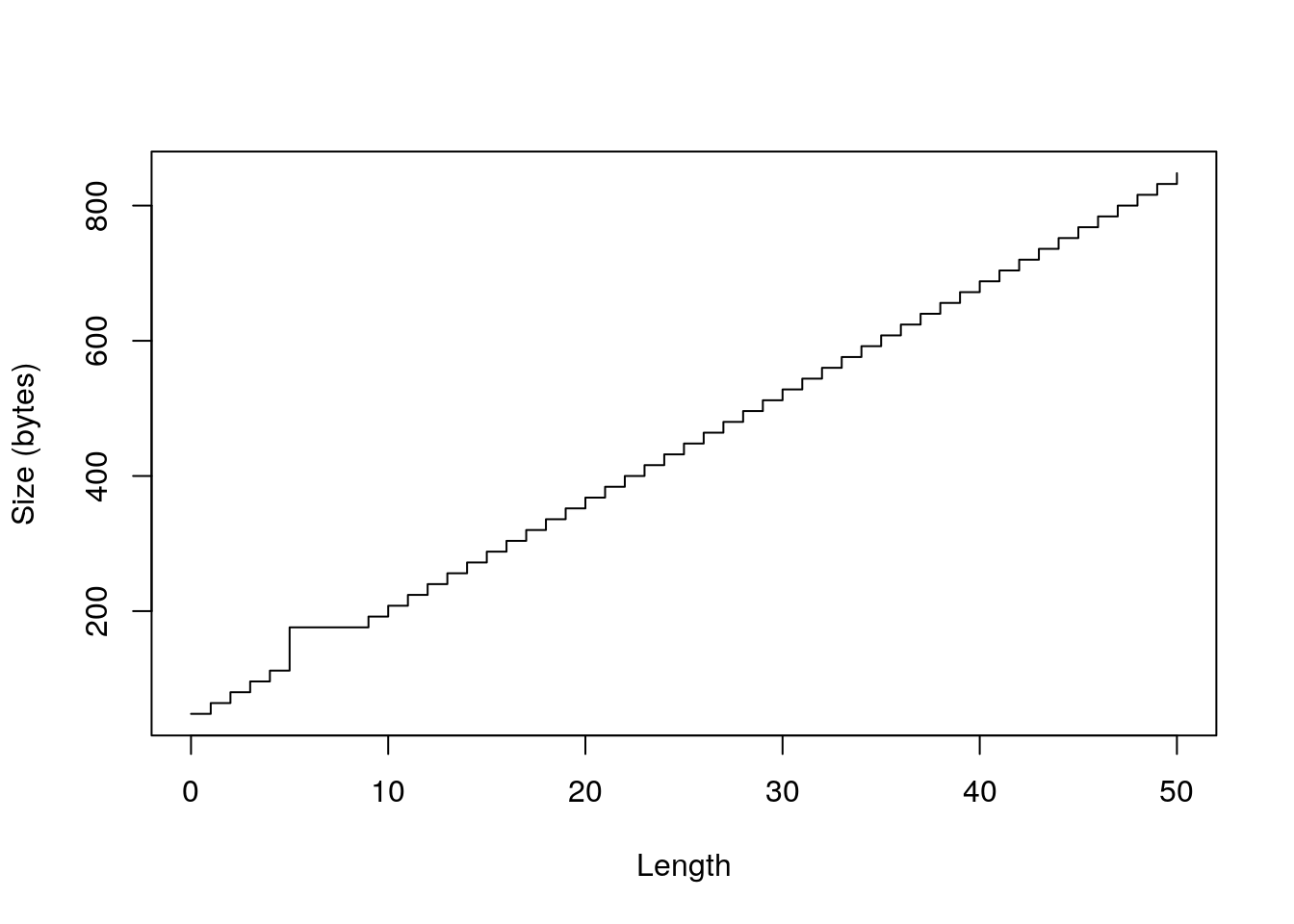plot(0:50, sizes - 40, xlab = "Length",
ylab = "Bytes excluding overhead", type = "n")
abline(h = 0, col = "grey80")
abline(h = c(8, 16, 32, 48, 64, 128), col = "grey80")
abline(a = 0, b = 16, col = "grey90", lwd = 4)
lines(sizes - 40, type = "s")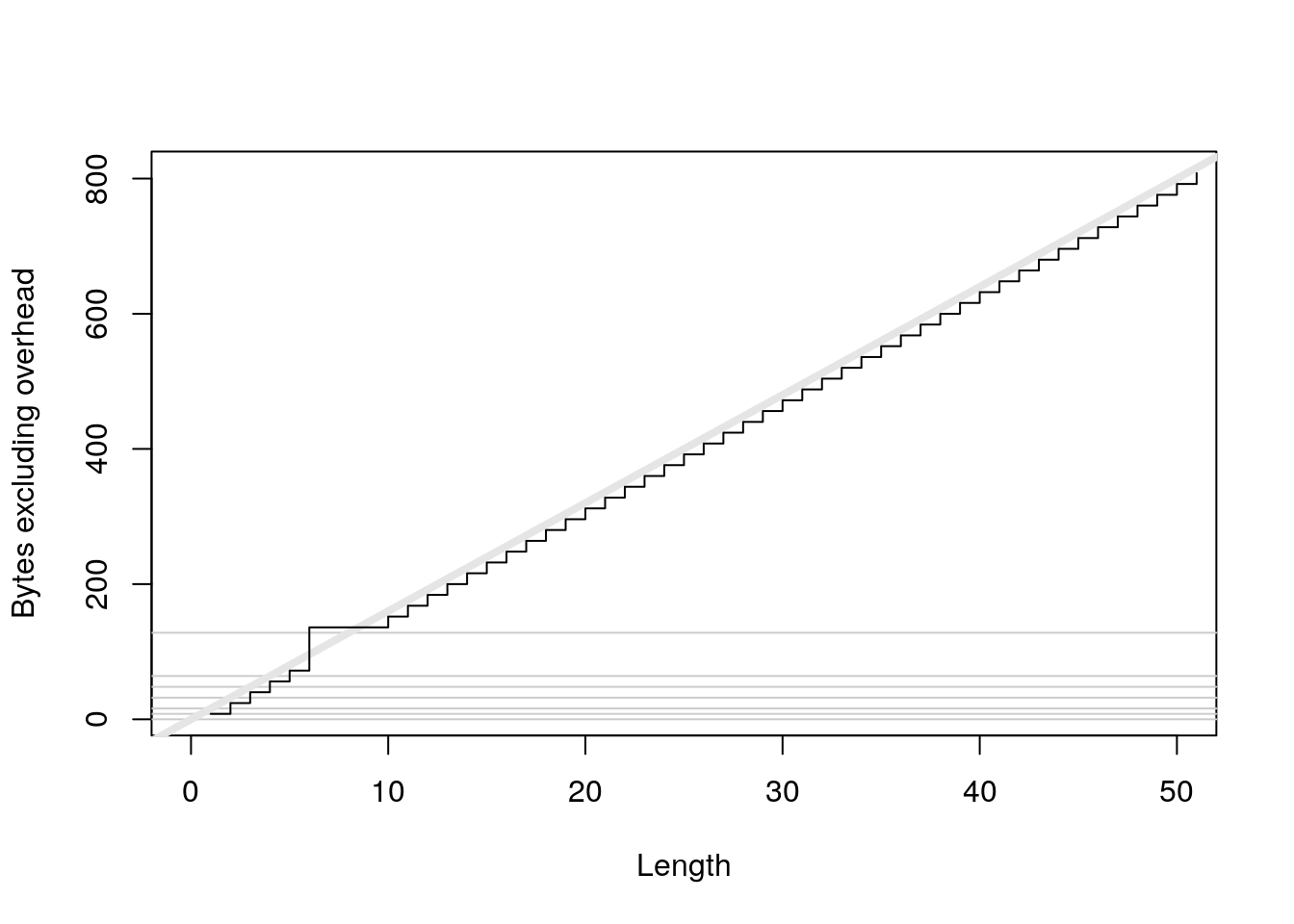x <- complex(1e6)
object_size(x)
#> 16 MB
y <- list(x, x, x)
object_size(y)
#> 16 MB
2. Q: If a data frame has one million rows, and three variables (two numeric, and one integer), how much space will it take up? Work it out from theory, then verify your work by creating a data frame and measuring its size.

A: From the textbook we know that

• an integer’s size is 40 bytes plus 4 bytes per allocated entry,
• a numerics’s size is 40 bytes plus 8 bytes per allocated entry.

So we can calculate the size via:

object_size(df) = 1 * (40 + 4 * 1,000,000) + 2 * (40 + 8 * 1,000,000) = 20,000,120 bytes.

And test this via:

df <- data.frame(int1 = integer(1000000),
num1 = numeric(1000000),
num2 = numeric(1000000))
as.integer(object_size(df))
#>  20000984

Note that we observe a small difference, because we didn’t include the costs for creating the data.frame() (560 bytes) in our previous calculations.

3. Q: Compare the sizes of the elements in the following two lists. Each contains basically the same data, but one contains vectors of small strings while the other contains a single long string.

vec <- lapply(0:50, function(i) c("ba", rep("na", i)))
str <- lapply(vec, paste0, collapse = "")

A:

vec <- lapply(0:50, function(i) c("ba", rep("na", i)))
str <- lapply(vec, paste0, collapse = "")
object_size(vec)
#> 13.9 kB
object_size(str)
#> 9.56 kB
object_size(vec, str)
#> 23.4 kB
4. Q: Which takes up more memory: a factor (x) or the equivalent character vector (as.character(x))? Why?

A: To be exact: it depends on the length of unique elements in relation to the overall length of the vector.

• In case of a long vector with only a few levels, the character takes approximately twice the memory of a factor:
object_size(rep(letters[1:20], 1000))
#> 161 kB
object_size(factor(rep(letters[1:20], 1000)))
#> 81.7 kB

That is, because a character allocates 8 bytes per entry (if the entry has less than 8 signs, otherwise roughly one byte per sign) and a factor equals an integer (allocates only 4 bytes per entry) with a character vector attribute that contains the levels (unique elements) of the vector:

a <- rep(1:20, 1000)
object_size(a)
#> 80 kB

attr(a, "levels") <- letters[1:20]
object_size(a)
#> 81.5 kB

class(a) <- "factor"
object_size(a)
#> 81.7 kB
• Of course the factor will allocate more memory, if all entries are unique:
object_size(letters[1:20])
#> 1.33 kB
object_size(factor(letters[1:20]))
#> 1.84 kB
5. Q: Explain the difference in size between 1:5 and list(1:5).

A: An empty list needs 40 bytes. For each entry 8 bytes are added. We can see this via:

object_size(vector("list",0))
#> 48 B
object_size(vector("list",1))
#> 56 B

Since 1:5 needs 72 bytes (note that for memory for short integers is allocated in chunks, as explained in the textbook), list(1:5) takes 120 bytes (48 + 72). So in general the cost for saving atomics within a list is 40 bytes for the list plus 8 bytes per atomic/list entry.

## 15.2 Memory profiling with lineprof

1. Q: When the input is a list, we can make a more efficient as.data.frame() by using special knowledge. A data frame is a list with class data.frame and row.names attribute. row.names is either a character vector or vector of sequential integers, stored in a special format created by .set_row_names(). This leads to an alternative as.data.frame():

to_df <- function(x) {
class(x) <- "data.frame"
attr(x, "row.names") <- .set_row_names(length(x[]))
x
}

What impact does this function have on read_delim()? What are the downsides of this function?

2. Q: Line profile the following function with torture = TRUE. What is surprising? Read the source code of rm() to figure out what’s going on.

f <- function(n = 1e5) {
x <- rep(1, n)
rm(x)
}

## 15.3 Modification in place

1. Q: The code below makes one duplication. Where does it occur and why? (Hint: look at refs(y).)

x <- data.frame(matrix(runif(100 * 1e4), ncol = 100))
medians <- vapply(x, median, numeric(1))
y <- as.list(x)
for(i in seq_along(medians)) {
y[[i]] <- y[[i]] - medians[i]
}

A: It occurs in the first iteration of the for loop. refs(y) is 2 before the for loop, because y is created via as.list(), which is not a primitive and so sets the refs counter up to two. Therefore R makes a copy, refs(y) becomes 1 and the following modifications will occur in place. The following code illustrates this behaviour, when run in the RGui. (Note that refs() will always return 2, when run in RStudio, as stated in the textbook. Note also, that you could detect this behaviour with the tracemem() function).

library(pryr)
rm(list = ls(all = TRUE))

x <- data.frame(matrix(runif(100 * 1e4), ncol = 4))
medians <- vapply(x, median, numeric(1))

y <- as.list(x)
is.primitive(as.list)
#  FALSE

for(i in seq_along(medians)) {
#  "0x11de30c8" "1" 
2. Q: The implementation of as.data.frame() in the previous section has one big downside. What is it and how could you avoid it?